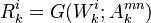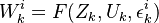# Conditional Independence

## Definition

Conditional Independence in the context of a Credit Network model is the adoption of a stochastic framework where the realisation of risk factors for individual entities of the network is independent once we condition on the realization of common (macro) factors

In more complicated credit contagion models, credit risk cascades are precipitated directly by default events

## Notation

In this class of model the rating state of each individual entity is completely determined by an implied credit quality indicator$R^i_k = G(W^i_k; A^{mn}_k)$$W^i_k = F(Z_k, U_k, \epsilon^i_k)$